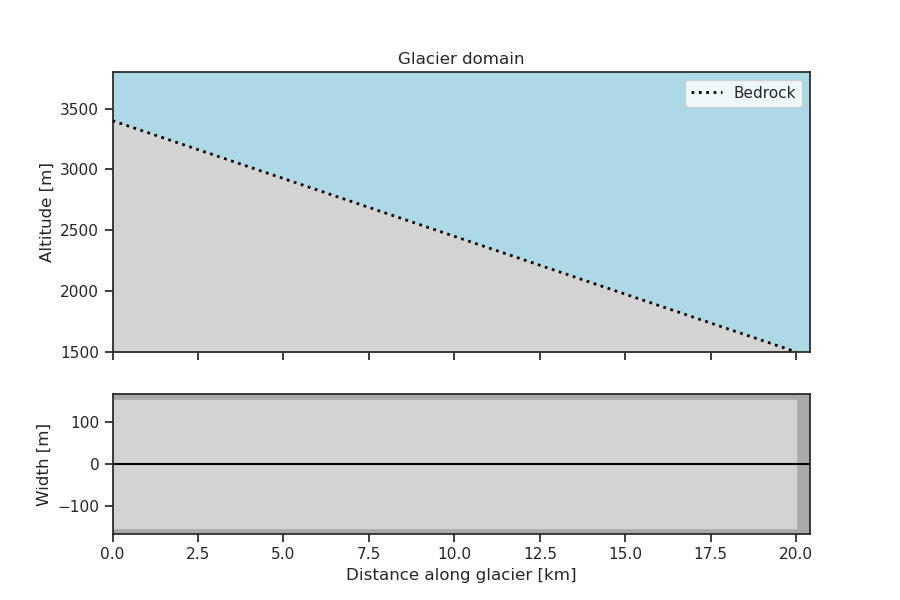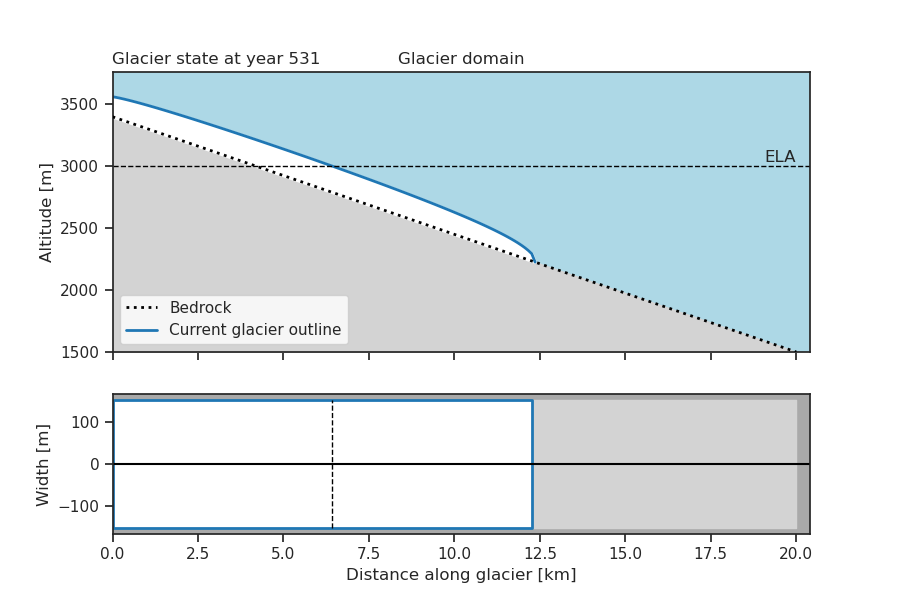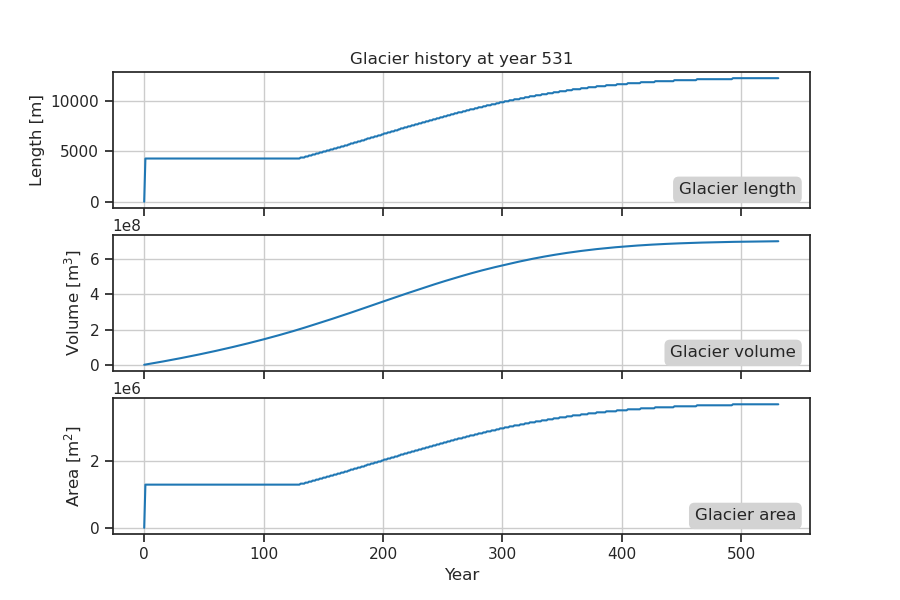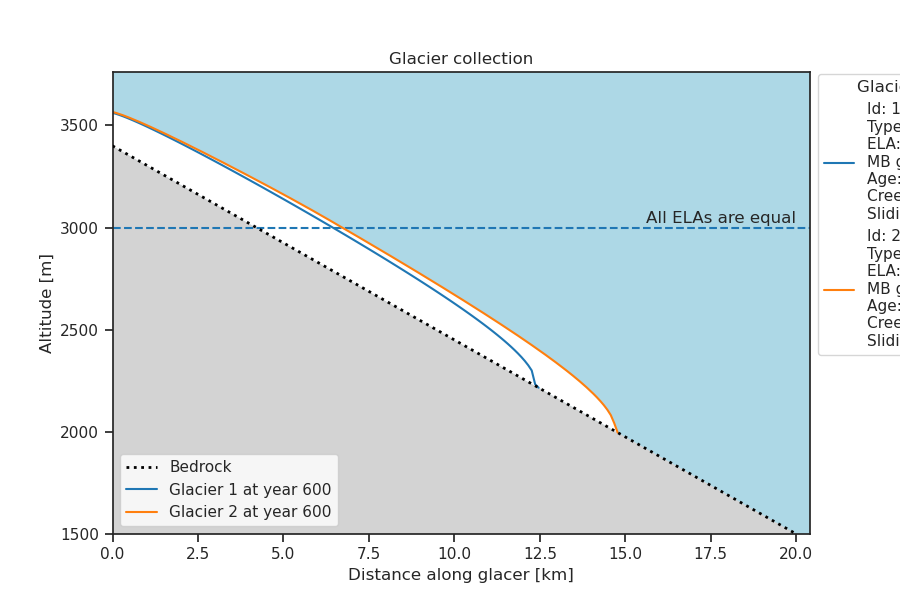# oggm-edu package documentation#

Here follows a list of the `oggm-edu` classes and their functionality. The best way to learn is to navigate between this static documentation and the rendered notebooks or the interactive version on MyBinder!## GlacierBed#

 `GlacierBed`([top, bottom, width, altitudes, ...]) The glacier bed used to construct a `oggm_edu.Glacier`. Plot the bed

### GlacierBed example#

```In : from oggm_edu import GlacierBed

In : bed = GlacierBed(top=3400, bottom=1500, width=300)

In : bed.plot();
```## MassBalance#

 `MassBalance`(ela, gradient[, breakpoints, ...]) Oggm Edu mass balance, used to construct the `oggm_edu.Glacier`. Reset the mass balance to initial state. `MassBalance.get_monthly_mb`(heights[, year]) Calculate the monthly mass balance for the glacier. `MassBalance.get_annual_mb`(heights[, year]) Get the annual mass balance. `MassBalance.gradient` Mass balance altitude gradient, mm/m. `MassBalance.ela` Altitude of the equilibrium line altitude (m). `MassBalance.temp_bias` Current temperature bias applied to the mass balance (unit: °C)

### MassBalance example#

```In : from oggm_edu import MassBalance

In : mass_balance = MassBalance(ela=3000, gradient=4)

In : mass_balance
Out:
Glacier mass balance
ELA [m]: 3000
Original ELA [m]: 3000
Temperature bias [C]: 0
```

## Glacier#

 `Glacier`(bed, mass_balance[, id]) Provides the user with an easy way to create and perform experiments on simulated glaciers.

### Glacier methods#

 Reset the glacier to initial state. `Glacier.copy`([id]) Return a copy of the glacier. Progress the glacier to year n. `Glacier.progress_to_equilibrium`([years, t_rate]) Progress the glacier to equilibrium. `Glacier.plot`([axes, title_number]) Plot the current state of the glacier. Plot the mass balance profile of the glacier. `Glacier.plot_history`([show_bias, window, ...]) Plot the history of the glacier. `Glacier.plot_state_history`([interval, eq_states]) Plot the state history of the glacier (thicknesses) at specified intervals. `Glacier.add_temperature_bias`(bias, duration) Add a gradual temperature bias to the future mass balance of the glacier.

### Glacier attributes#

 `Glacier.ela` Expose the mass balance ela for the glacier. `Glacier.mb_gradient` Expose the mass balance gradient. `Glacier.mass_balance` Glacier mass balance. `Glacier.annual_mass_balance` The annual mass balance at each grid point of the glacier. `Glacier.specific_mass_balance` The specific mass balance of the glacier [m w.e. `Glacier.age` Set the age of the glacier. `Glacier.history` The history of the glacier. `Glacier.state_history` The state history of the glacier, i.e. geometrical changes over time. `Glacier.creep` Set the value for the creep parameter A in Glens equation. `Glacier.basal_sliding` Set the sliding parameter of the glacier `Glacier.eq_states` Glacier equilibrium states. `Glacier.response_time` The response time of the glacier. `Glacier.current_state` For advanced users: get access to the current OGGM `Flowline` object.

### Glacier example#

```In : from oggm_edu import Glacier

In : glacier = Glacier(bed=bed, mass_balance=mass_balance)

In : glacier.progress_to_equilibrium()

In : glacier.plot();
``````In : glacier.plot_history();
```## SurgingGlacier#

 `SurgingGlacier`([bed, mass_balance]) A surging glacier. Reset the state of the surging glacier. Progress the surging glacier to specified year. Plot the history of the surging glacier. `SurgingGlacier.normal_years` Number of years that the glacier progress without surging. `SurgingGlacier.surging_years` Number of years that the glacier progress during surges. `SurgingGlacier.basal_sliding_surge`

## GlacierCollection#

 `GlacierCollection`([glacier_list]) This is an object used to store multiple glaciers.

### GlacierCollection methods#

 Reset all glaciers in the collection `GlacierCollection.fill`(glacier, n[, ...]) Fill the collection with a desired number of glaciers. `GlacierCollection.add`(glacier) Adds one or more glaciers to the collection. Change the attribute(s) of the glaciers in the collection. Progress the glaciers within the collection to the specified year. Progress the glaciers to equilibrium. Plot the glaciers in the collection to compare them. Plot the collection but side by side. Plot the histories of the collection. Plot the mass balance(s) for the glaciers in the collection.

### GlacierCollection attributes#

 `GlacierCollection.glaciers` Glaciers stored in the collection `GlacierCollection.annual_mass_balance` Glaciers mass balances

### GlacierCollection example#

```In : from oggm_edu import GlacierCollection

In : wide_narrow_bed = GlacierBed(altitudes=[3400, 2800, 1500],
....:                              widths=[600, 300, 300])
....:

In : wide_narrow_glacier = Glacier(bed=wide_narrow_bed,
....:                               mass_balance=mass_balance)
....:

In : collection = GlacierCollection()